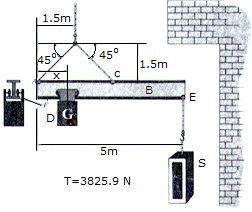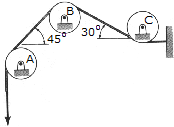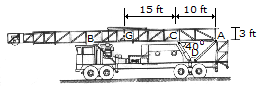# Engineering Mechanics - Equilibrium of a Rigid Body

### Exercise :: Equilibrium of a Rigid Body - General Questions

• Equilibrium of a Rigid Body - General Questions
11.If the boom in the previous problem is to remain horizontal when the stone S is removed, what is x?

 A. x = 1.180 m B. x = 2.500 m C. x = 0.340 m D. x = 0.660 m

Explanation:

No answer description available for this question. Let us discuss.

12.Compute the horizontal and vertical components of force at pin B. The belt is subjected to a tension of T=100 N and passes over each of the three pulleys.

 A. Bx = 0 N, By = 141.4 N B. Bx = -15.89 N, By = 120.7 N C. Bx = 20.7 N, By = 157.3 N D. Bx = 0, By = 100.0 N

Explanation:

No answer description available for this question. Let us discuss.

13.The oil rig is supported on the trailer by the pin or axle at A and the frame at B. If the rig has a weight of 115,000 lb and the center of gravity at G, determine the force F that must de developed along the hydraulic cylinder CD in order to start lifting the rig (slowly) off B toward the vertical. Also compute the horizontal and vertical components of reaction at the pin A.

 A. Ax = 343 kip, Ay = -172.5 kip, FCD = 447 kip B. Ax = 205 kip, Ay = -57.5 kip, FCD = 268 kip C. Ax = 241 kip, Ay = -172.5 kip, FCD = 375 kip D. Ax = 220 kip, Ay = -70.1 kip, FCD = 288 kip